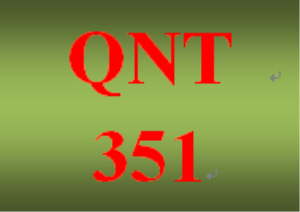# QNT 351 Week 3 Connect Problem Set

PLDZ-4 In Stock
\$ 10.00 USD
Description

https://uopcourses.com/category/qnt-351/

1.

A sample of 43 observations is selected from a normal population. The sample mean is 30, and the population standard deviation is 3. Conduct the following test of hypothesis using the 0.05 significance level.
H0 : μ ≤ 29
H1 : μ > 29
a. Is this a one- or two-tailed test?
b. What is the decision rule? (Round your answer to 3 decimal places.)
c. What is the value of the test statistic? (Round your answer to 2 decimal places.)
d,
What is your decision regarding H0?

e. What is the p-value? (Round your answer to 4 decimal places.)p-value

2.At the time she was hired as a server at the Grumney Family Restaurant, Beth Brigden was told, “You can average \$86 a day in tips.” Assume the population of daily tips is normally distributed with a standard deviation of \$3.81. Over the first 48 days she was employed at the restaurant, the mean daily amount of her tips was \$87.07. At the 0.02 significance level, can Ms. Brigden conclude that her daily tips average more than \$86?

a. State the null hypothesis and the alternate hypothesis.
b. State the decision rule.
c. Compute the value of the test statistic. (Round your answer to 2 decimal places.)
Value of the test statistic
d. What is your decision regarding H0?
e. What is the p-value? (Round your answer to 4 decimal places.)

3.
The Rocky Mountain district sales manager of Rath Publishing Inc., a college textbook publishing company, claims that the sales representatives make an average of 43 sales calls per week on professors. Several reps say that this estimate is too low. To investigate, a random sample of 41 sales representatives reveals that the mean number of calls made last week was 44. The standard deviation of the sample is 2.9 calls. Using the 0.100 significance level, can we conclude that the mean number of calls per salesperson per week is more than 43?

H0 : μ ≤ 43
H1 : μ > 43

1. Compute the value of the test statistic. (Round your answer to 3 decimal places.)

Value of the test statistic

2. What is your decision regarding H0?

4.
A United Nations report shows the mean family income for Mexican migrants to the United States is \$27,150 per year. A FLOC (Farm Labor Organizing Committee) evaluation of 30 Mexican family units reveals a mean to be \$29,500 with a sample standard deviation of \$11,150. Does this information disagree with the United Nations report? Apply the 0.01 significance level.

a. State the null hypothesis and the alternate hypothesis.
b. State the decision rule for .01 significance level. (Negative amounts should be indicated by a minus sign. Round your answers to 3 decimal places.)
c. Compute the value of the test statistic. (Round your answer to 2 decimal places.)

Value of the test statistic
d. Does this information disagree with the United Nations report? Apply the 0.01 significance level.

5.
The following information is available.
H0 : μ ≥ 220
H1 : μ < 220
A sample of 64 observations is selected from a normal population. The sample mean is 215, and the population standard deviation is 15. Conduct the following test of hypothesis using the .025 significance level.
a. Is this a one- or two-tailed test?
b. What is the decision rule? (Negative amount should be indicated by a minus sign. Round your answer to 2 decimal places.)
c. What is the value of the test statistic? (Negative amount should be indicated by a minus sign. Round your answer to 3 decimal places.)
d. What is your decision regarding H0?
e. What is the p-value? (Round your answer to 4 decimal places.)
6.
Given the following hypotheses:
H0 : μ ≤ 10
H1 : μ > 10
A random sample of 10 observations is selected from a normal population. The sample mean was 12 and the sample standard deviation 3. Using the .05 significance level:

a. State the decision rule. (Round your answer to 3 decimal places.)

Reject H0 if t >
b. Compute the value of the test statistic. (Round your answer to 3 decimal places.)
Value of the test statistic

c. What is your decision regarding the null hypothesis?

Given the following hypotheses:

H0 : μ = 400

H1 : μ ≠ 400

A random sample of 12 observations is selected from a normal population. The sample mean was 407 and the sample standard deviation 6. Using the .01 significance level:

a. State the decision rule. (Negative amount should be indicated by a minus sign. Round your answers to 3 decimal places.)

b. Compute the value of the test statistic. (Round your answer to 3 decimal places.)

cWhat is your decision regarding the null hypothesis?"

Recent Reviews Write a Review
0 0 0 0 reviews